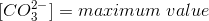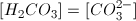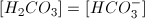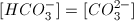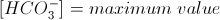# MCAT Physical : Titration Curves

## Example Questions

### Example Question #1 : Titration Curves

Consider the titration curve, in which an acid is treated with aqueous 0.5M NaOH.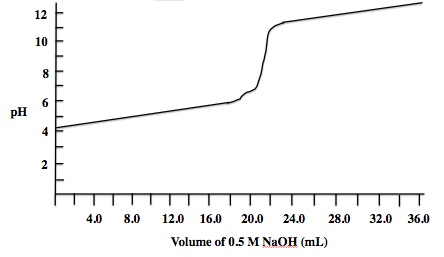Which of the following compounds could be the acid that is being titrated in this experiment?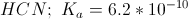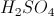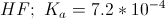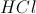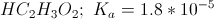Explanation:

NaOH is a strong base, so if the acid were a strong acid, the pH at equivalence would be 7. The graph, however, shows that the pH at equivalence is greater than 7, which means the acid being titrated is a weak acid.

We can also find the approximate pKa value from the half equivalence point. In the graph, the equivalence point occurs at a pH around 8.9, corresponding to the addition of about 22mL of NaOH. The half equivalence point will thus be after the addition of approximately 11mL of NaOH, giving a pH of 5.0. The pH at the half equivalence is equal to the pKa, meaning that the Ka value must be around 10-5.

This leads to our answer,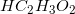.

### Example Question #1 : Titration Curves

A titration reaches its equivalence point. You measure the pH and find it to be 6.4. You were most likely working with which of the following sets of compounds:

HF and KOH

HCN and LiOH

HCl and NaOH

H2S and C5H8O2

HNO3 and NH3

HNO3 and NH3

Explanation:

For a strong acids and strong base, the equivalence pH will be 7.0. We can see that our equivalence pH is less than 7.0, meaning that the acidic component is prevailing. Hence, we must be working with an acid that is stronger than our base. HNO3 is a strong acid, and NH3 is a weak base, meaning that the acid will prevail, and the pH will be acidic (<7.0).

The other answer choices show a strong acid and strong base (HCl and NaOH), a weak acid and strong base (HF and KOH, HCN and LiOH), and a weak acid and weak base (H2S and C5H8O2). Though the equivalence pH of a weak acid and weak base may not be 7.0, we do not have enough information to choose this answer. The best answer choice is HNO3 and NH3

### Example Question #1 : Titrations

Which of the following pH values is an acceptable equivalence point for a weak base being titrated by a strong acid?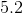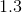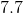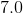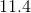Explanation:

The equivalence point is the point during a titration when there are equal equivalents of acid and base in the solution. Since a strong acid will have more effect on the pH than the same amount of a weak base, we predict that the solution's pH will be acidic at the equivalence point. 5.2 and 1.3 are both acidic, but 1.3 is remarkably acidic considering that there is an equal amount of base in the solution. As a result, 5.2 is a more appropriate answer.

The equivalence point for a strong acid and strong base will be 7.0. When one of the compounds is weak, however, it will dissociate less than its strong counterpart. In our case, the base will dissociate less than the acid. The acid, thus, contributes more to the pH character of the solution.

### Example Question #1 : Titration Curves

A weak acid is slowly titrated with a strong base. Where on the titration curve would the solution be the most well-buffered?

When the amount of base added equals the amount of acid in the solution.

The equivalence point

When no base has been added

The half equivalence point

The half equivalence point

Explanation:

At the half equivalence point, the concentration of acid in the solution is equal to the concentration of the conjugate base in solution. At this point, the graph shows a line that is near horizontal. This means that base or acid could be added and the pH of the solution would change very slowly.

Remember that pH is on the y-axis of the titration curve; thus a near-horizontal line will signify a region where pH is most stable. At the half equivalence point, pH = pKa.

### Example Question #2 : Titrations

Which of the following curves represents the titration of a strong base by a strong acid?

Explanation:

Since we are adding acid to a base, the pH must decrease. The initial base will have a high pH, while the final acid will have a low pH; the right of the curve must be lower than the left. In addition, the pH does not change very rapidly until the equivalence point is reached, hence the curve must show little initial change followed by a rapid change.

### Example Question #3 : Titration Curves

Acids and bases can be described in three principal ways. The Arrhenius definition is the most restrictive. It limits acids and bases to species that donate protons and hydroxide ions in solution, respectively. Examples of such acids include HCl and HBr, while KOH and NaOH are examples of bases. When in aqueous solution, these acids proceed to an equilibrium state through a dissociation reaction.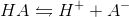All of the bases proceed in a similar fashion.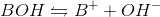The Brønsted-Lowry definition of an acid is a more inclusive approach. All Arrhenius acids and bases are also Brønsted-Lowry acids and bases, but the converse is not true. Brønsted-Lowry acids still reach equilibrium through the same dissociation reaction as Arrhenius acids, but the acid character is defined by different parameters. The Brønsted-Lowry definition considers bases to be hydroxide donors, like the Arrhenius definition, but also includes conjugate bases such as the A- in the above reaction. In the reverse reaction, A- accepts the proton to regenerate HA. The Brønsted-Lowry definition thus defines bases as proton acceptors, and acids as proton donors.

A scientist decides to study an acid,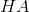, by carefully adding a base to the solution through a titration. At the equivalence point of an acid-base titration __________.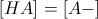half of the acid has been neutralized by the titrant

all the acid has been neutralized by the titrant

all the base has been neutralized by the titrant

half of the base has been neutralized by the titrant

all the acid has been neutralized by the titrant

Explanation:

A titrant is added to a solution being studied. In this scenario, the base is the titrant and the equivalence point is reached when all of the acid has been neutralized by the base. The equivalence point is defined as the point at which moles of added titrant equal the moles of initial solute in solution.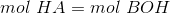The equimolar concentration of acid and conjugate base happens at a point known as the half-equivalence point, which can be confusing.

### Example Question #21 : Acid Base Chemistry

Consider the titration curve, in which an acid is treated with aqueous 0.5M NaOH.The pH at the equivalence point was found to be 8.9. Approximately how many milliliters of 0.5M NaOH were needed to reach equivalence?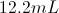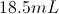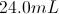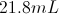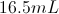Explanation:

For any titration curve the equivalence point corresponds to the steepest part of the curve. In this case, the question indicates that the pH at equivalence was 8.9, which would correspond to a volume between 20.0 and 24.0 on the x-axis of the graph. This leads to our approximate answer of 21.8mL.

### Example Question #21 : Analytical Chemistry

Consider the titration curve, in which an acid is treated with aqueous 0.5M NaOH.What is the approximate pKa of the starting acid?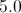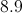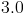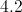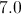Explanation:

The pKa corresponds to the pH when half of the acid has been neutralized. In this case, that would correspond to when half of the 0.5M NaOH has been added. We can see in the curve that the equivalence point is at around 22mL of NaOH, as this is the steepest change in pH. The half equivalence point will be found at approximately 11mL of NaOH addition. On the graph, 11mL of NaOH results in a pH of approximately 5.0, giving us our pKa value.

### Example Question #23 : Acid Base Chemistry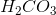is a diprotic acid. Which of the following statements is true about its second half equivalence point?